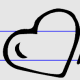Home > Nanette Johnson (page 4)

## Nanette Johnson## Multiply to Make -64

Directions: Find three numbers whose product is -64. You may use integers from -10 to 10. You may not use the same absolute value twice. Find all possible combinations. Source: Nathan Charlton and Daniel Martinez

## Angles of a Polygon

Directions: The measures of the angles of a convex polygon form an arithmetic sequence. The smallest angle has a measurement of 129 degrees. The largest angle has a measurement of 159 degrees. Find the number of sides in this polygon. Source: Ricardo Navarro

## Subtraction to Get the Smallest Difference

Directions: Using the digits 1 to 9, at most one time each, fill the boxes below to create the smallest possible difference. Source: Graham Fletcher

## Equality

Directions: Using the digits 0-9 at most one time each, fill in the boxes below to make a true statement. Source: Graham Fletcher

## Equivalent Equations

Source: Smarter Balance 7th grade practice test

## How Many Squares?

Directions: How many squares are shown in the diagram below? Source:  This problem was found in the Fifth Edition Elementary Geometry for College Students by Alexander and Koeberlein

## Equation of the Biggest Circle

Directions: Using any integers, fill in the blanks to Fill in the blanks with integers so that: The equation’s graph is a circle. The circle has the biggest area The circle is completely inside the first quadrant The circle’s radius is a whole number 1 through 9. Source: Nanette Johnson

## Is the Quadrilateral a Kite?

Directions: What is the least number of geometric markings to show that a quadrilateral is a kite? Source: Nanette Johnson